# Equivalent Circuits Of Single Phase Transformers Electrical Engineering (EE) Notes | EduRev

## Electrical Engineering (EE) : Equivalent Circuits Of Single Phase Transformers Electrical Engineering (EE) Notes | EduRev

The document Equivalent Circuits Of Single Phase Transformers Electrical Engineering (EE) Notes | EduRev is a part of the Electrical Engineering (EE) Course Electrical Machines.
All you need of Electrical Engineering (EE) at this link: Electrical Engineering (EE)

Equivalent Circuits of Single Phase Transformers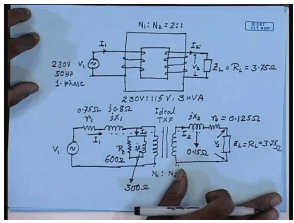In the last lecture, we have seen that a practical single phase transformer like this supplied from a single phase constant frequency source, and connected to a load on the other side drawing current I 1 from the primary and supplying a current I 2, on the secondary can be modeled using an ideal transformer along with other passive components that represents the non-ideality of the transformer. This is the ideal transformer. Non idealities to be included are a core loss resistance, a magnetizing resistance, the leakage reactants in the primary site and the winding resistance of the primary site; similarly, the leakage reactants on the secondary site and the winding resistance of the secondary site.
This is the load; this is the output voltage V 2. This is secondary resistance R 2; this is secondary leakage reactants j x 2, the primary leakage reactants j x 1, primary winding resistance R 1, core loss resistance R 0, magnetizing reactants j x m. So, it draws it is connected to a constant voltage constant frequency source V 1 draws a current I 1 from the primary site, and no load current is I 0. It sends out a current I 2 on the secondary side; the turns ratio being N 1 is to N 2. This is the model of a practical single phase transformer incorporating an ideal transformer; this is the ideal transformer.

We have also mentioned that this model is not very convenient for applying all the circuit analyses tools that we have learnt in our electrical technology class. Since, there is a isolation barrier at the ideal transformer which means we cannot for example, choose a loop current across the primary and secondary; since, we do not know the voltage drop between the primary and the secondary winding of the ideal transformer. Therefore, we said that this can be converted to a convenient equivalent circuit by either referring whatever is there on the secondary site to the primary or referring the primary to the secondary site which results in two equivalent circuit which are very closely related as follows. The equivalent circuit referred to primary will look like this.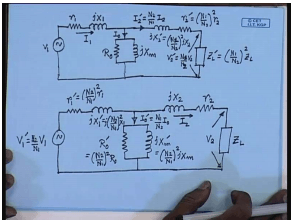The elements on the primary side do not change so that they retain their value; however, the elements on the secondary side has to be referred to primary with a given turns ratio. So, primary voltage will still be V 1, primary winding resistance will be R 1, leakage reactants will be j x 1, core loss resistance and magnetizing reactants are also generally referred to the primary. So, this will remain R 0, this will be j x m. The primary current will be I 1, no load current I 0. The secondary current I 2 will now be replaced by I 2 dashed given by N 2 by N 1 into I 2. The secondary leakage reactants j x 2 dash is given by N 1 by N 2 square j x 2.
Similarly, the referred secondary winding resistance R 2 dash will be given by N 1 by N 2 square R 2. The load impedance Z L dash the referred loading impedance Z L dash will be given by N 1 by N 2 square Z L, and the output voltage referred output voltage V 2 will be V 2 dash will be N 1 by N 2 into V 2 . So, this is the exact equivalent circuit, why we are using the term exact we will come later on, of the single phase transformer referred to the primary side. Of course, this can be this equivalent circuit can be drawn referred to the secondary side as well, and that circuit also we have shown the other day. It is in that case the secondary elements are not changed, but all the primary elements are referred to the secondary by the trans ratio.

Now the secondary load impedance Z L remain as it is, winding resistance remain as it is, leakage reactants remains as it is, the current I remains I 2, the load voltage remain v two; however, the primary side elements for example, the magnetizing reactants now j x m dash will be equal to N 2 by N 1 whole square j x m. R 0 dash will be equal to N 2 by N 1 whole square R 0. I 0 dash will be equal to N 1 by N 2 into I 0. The primary winding resistance R 1 dash will be equal to N 2 by N 1 whole square R 1. Primary leakage reactants j x 1 dash will be given by N 2 by N 1 whole square x 1, and the source voltage V 1 dash will be equal to N 2 by N 1 V 1. So, these are the two equivalent circuits.

The first one is referred to the primary with number of turns N 1; the second one is referred to the secondary with number of turns N 2. Now the major application of this equivalent circuits are in determining the different variables; for example, if V 1 is given, the load parameter is given, and the circuit parameter is given, then you can find out all the currents, the voltage, etcetera. Let us look at a concrete example. Let us say that this is a single phase transformer. The ratings are 230 volt is to 115 volt, and the KVA rating is 23 KVA which means N 1 is to N 2 for this case is 2 is to 1. Also let us say the load impedance is actually a load resistance of value 3.75 ohm.

The other parameters R 1, say, it is given as 0.75 ohm. X 1 let us say it is j 0.8 ohm. R 0 given to be 600 ohm; j x m is given to be 300 ohm. Similarly x 2 dash, x 2 rather is given to be 0.15 ohm and R 2 is given as 0.125 ohm. The problem is to find out what will be the load terminal voltage V 2, what will be the load current I 2, what will be the current drawn from the source I 1 and what will be the no load current I 0? How are you going to solve this problem? So, as a first step let us draw the equivalent circuit referred to primary; the source voltage is given to be 230 volt 50 hertz single phase.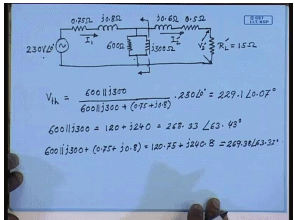So, let us draw the equivalent circuit of the transformer referred to primary 0.18 ohm. Since we have referred it to the primary it will be j X L 2 x 2 dash and its value will be 0.6. Since the actual value referred to the secondary was 0.15 ohm, and N 1 by N 2 is 2; therefore, x 2 dash will be 0.15 into N 1 by N 2 square which is 4 and hence 0.6 ohm. Similarly R 2 dashed will be 0.5 ohm, and R L dash will be equal to 15 ohm. The output voltage is V 2 dash; output current is I 2 dash; input current is I 1; input voltage is 230 volt 50 hertz. So, let us take it to be our reference pressure at 0 degree. The magnetization branch is referred to the primary. So, these values do not change. This is 600 ohm; this is j 300 ohm. Now there are different ways of solving this circuit.

For example, the loop current method or the node voltage method, however, for this particular problem we will apply the Thevenin’s theorem. So, let us find out the Thevenin equivalent circuit at these two terminals looking back. If v Thevenin at these two terminal is given by impedance here divided by the total impedance into the voltage; that is 600 parallel j 300 divided by 600 parallel j 300 plus 0.75 plus j0.8 into 230 angle 0 degree. Now 600 parallel j 300 is equal to 120 plus j 240; similarly, the denominator becomes 600 parallel j 300 plus 0.75 plus j 0.8 becomes 120.75 plus j 240.8 which is equal to 269.38 angle 63.37 degrees. And this is 268.33 angle 63.43 degrees, and V t h is if we calculate comes to be 229.1 angle 0.07 degrees. So, what is Z t h? Z t h will be the equivalent impedance seen at this terminal when the input source is sorted.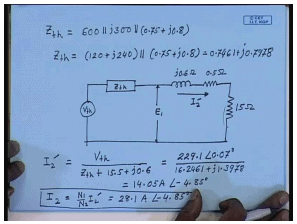Therefore Z t h is equal to 600 parallel j 300 parallel 0.75 plus j 0.8. In other words Z t h equal to 120 plus j 240 parallel with 0.75 plus j 0.8, and if this is computed Z t h comes to be 0.7461 plus j 0.7978. So, what will be I 2 dash in this circuit? I 2 dashed will be; so what is the Thevenin equivalent circuit let us first see. The Thevenin equivalent circuit becomes V t h in series with Z t h in series with rest of the circuit. Please note that if this voltage in the actual circuit was E 1 the voltage at this terminal will also be E 1. Since E 1 equal to I 2 dashed into this complete impedance here also E 1 equal to I 2 dashed into this impedance, and this impedance do not change because only the input side has been changed.

So, what will be I 2 dash? I 2 dash will be equal to v Thevenin divided by z Thevenin plus sum of these impedances which is 15.5 plus j0.6. This will be equal to 229.1 angle 0.07 degrees; thats what we have found for V t h previously, and the total impedance will be 16.2461 plus j1.3978, and this comes to be 14.05 amperes at an angle of minus 4.85 degree with respect to the primary applied voltage. Now I 2, how much is I 2? I 2 equal to N 1 by N 2 into I 2 dash; hence, I 2 is 28.1 ampere at an angle of minus 4.85 degree with respect to the input applied voltage. Therefore, we have found answer to one of the problems; that is we have found what is the output current? So, what is the output voltage? For that we will calculate what is V 2 dash? V 2 dash is nothing but I 2 dash into load resistance.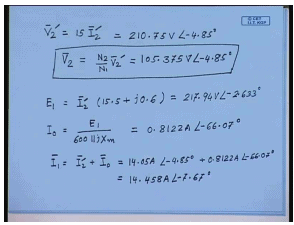And this comes to 210.75 volts at an angle of minus 4.85 degrees with respect to the input applied voltage. What is V 2? The actual load voltage V 2 equal to N 2 by N 1 into V 2 dash; hence it is, 105.375 volt angle minus 4.85 degrees. So, this is the answer to the second part of our question what is the load voltage. How do we find out the magnetizing current? Please note that we have already argued that if the voltage at the air gap, this is for the air gap voltage is E 1, in the actual equivalent circuit then in this Thevenin equivalent circuit also the voltage will be E 1. So, let us try to find out what is E 1 first. Now E 1 from this Thevenin equivalent circuit is I 2 dashed multiplied by the total impedance of the secondary side. These are all phasors.

And if we calculate this comes to 217.94 volts at an angle of minus 2.633 degrees with respect to the primary applied voltage v 1. So, what is no load current I 0? I 0 is E 1 divided by 600 parallel j x m that is from this actual equivalent circuit. Hence if we compute I 0 will come to be 0.8122 ampere at an angle of minus 66.07 degrees. This is phase angle with respect to the primary applied voltage V 1; since that is what we have assumed to be our reference phasor with angle 0. What is I 1 then? Obviously, I 1 is the phasor sum of I 2 dashed and I 0. It should be noted that the current supplied by the Thevenin source is not I 1; it is I 2 dash.

So, therefore, to find out the source current I 1 we should go back to the original equivalent circuit where before we took the Thevenin equivalent circuit. Therefore I 1 is given by I 2 dash plus I 0, phasor sum of I 2 dashed and I 0; that is we have found out I 2 dashed to be 14.05 ampere angle minus 4.85 degrees, and I 0 to be 0.8122 ampere angle at minus 66.07 degrees. Therefore, if we do the computation I 1 will come as 14.458 amperes angle minus 7.67 degrees. So, that shows how to use this equivalent circuit to compute different variables voltages and currents at different parts of the transformer. And it will be interesting to solve the same problem using this equivalent circuit referred to the secondary, but this is left as an exercise.

However, the purpose of solving this problem was not just to show how this problem can be solved. I would like to draw your attention to a few salient features of this equivalent circuit. For example, let us see the applied voltage; the applied voltage was 230 volt at an angle of 0 degree while when we computed the Thevinin voltage it comes to something like 229.1 volt angle 0.07 degrees. Now is this very different from the actual applied voltage? Similarly the Thevinin impedance Z t h comes to 0.7461 plus j 0.7978. If you look at the actual primary impedance it was 0.75 ohm and 0.8 ohm here; therefore, it possibly make sense in order to reduce the computational complexity to obtain this thevinin equivalent circuit by simply omitting this part and connecting this right across the supply voltage.

In fact, that is what is done in most practical cases; since, the results that are obtained by that approximation is not very different from this exact equivalent circuit; we will shortly see that. The rational is this impedance of the magnetizing branch being much higher than the impedance of the primary winding; that is winding resistance and leakage reactants, the inter applied primary voltage appears almost across the magnetization branch. The drop across the primary impedance is very small even at a rated current; with that assumption the exact equivalent circuit referred to the primary can be simplified by the following approximate equivalent circuit.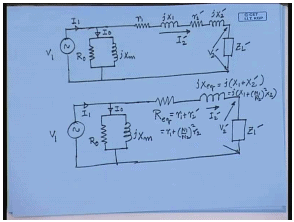Here in the approximate equivalent circuit the magnetization branch is connected right across the source, and the primary impedance and secondary impedance are connected together V 2 dash load current I 2 dash. Please note this was the exact equivalent circuit. In order to get the approximate equivalent circuit what I have done I have simply shifted this magnetization branch from this middle point to the beginning that is right across the source to get the approximate equivalent circuit. The underlined assumption being the drop across the across the primary winding impedance is very small compared to the total applied voltage. Now this can be further simplified under secondary the resistances can be clubbed together, and reactance’s can be clubbed together to give a even simpler form of the semi equivalent circuit.

It is equal to R 1 plus N 1 by N 2 square R 2, and j x e q equal to j x 1 plus x 2 dash; that is equal to j x 1 plus N 1 by N 2 square x 2. This remains Z L dash, current is I 2 dash is equal to this V 2 dash. The primary current is I 1, magnetizing current is the no load current is I 0; obviously, this circuit this approximate equivalent circuit is far simpler compared to the exact equivalent circuit that has been shown earlier. Now how much error do we incur if we use the approximate equivalent circuit instead of the actual equivalent circuit? Let us try; let us try with the same problem.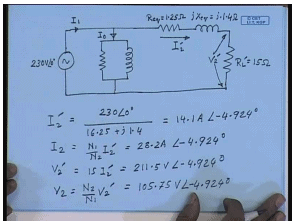So, the approximate equivalent circuit for the earlier problem will be this is 230 volt angle 0 degree. We have to find out this current I 1, the no load current I 0. R e q from the given data is equal to 1.25 ohm; X e q from the given data is j1.4 ohm. The load resistance R L dash equal to 15 ohm. This is referred load voltage V 2 dash; this is the referred load current I 2 dashed. So, how do we compute I 2 dashed here? I 2 dashed is simply equal to 230 angle 0 degree divided by 16.25 sum of these impedances plus j1.4. This is given by 14.1 ampere angle minus 4.924 degrees.

So, I 2 equal to N 1 by N 2 I 2 dash is 28.2 ampere angle minus 4.924 degrees. What is V 2 dash? V 2 dashed again is 15 I 2 dash, and the value is 211.5 volt angle minus 4.924 degrees, and V 2 equal to N 2 by N 1 V 2 dash equal to 105.75 volts angle minus 4.92 degrees. The simplification in the calculation is very apparent; we do not have to find out the Thevenin equivalent voltage or Thevenin impedance. We can simply calculate the variance.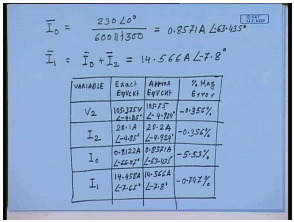I 0 and I 1 can be computed; similarly, I 0 equal to 230 angle 0 degree divided by 600 parallel j 300, and the value is 0.8571 amperes at an angle of minus 63.435 degree with respect to the applied voltage phasor. The input current I 1 is the phasor sum of I 0 plus I 2 dash and after computation if value comes to 14.566 ampere angle at minus 7.8 degrees. So, the amount of simplification in computation is very apparent. So, let us compare the accuracy. So, this we can do by drawing this table. So, let us see; this is the variable name. Next let us put the exact value from the exact equivalent circuit. Then value from the approximate equivalent circuit and percentage error let us say only in magnitude. F
First variable let us take the load voltage V 2. The value you have got by exact equivalent circuit is 105.375 volt at an angle of minus 4.85 degrees, and from the approximate equivalent circuit we have got it to be 105.75 volts at an angle of minus 4.924 degrees. The percentage error in magnitude which is this value minus this value divided by this comes to minus 0.356 percent. For I 2 magnitude was 28.1 ampere, angle was minus 4.85 degrees. Here the magnitude was 28.2 amperes, angle was minus 4.924 degrees. Again the percentage error in magnitude is just 0.356 percent. What about I 0? The magnitude by exact equivalent circuit came to be 0.8122 ampere at an angle of minus 66.07 degrees.
By approximate equivalent circuit it came to 0.8571 amperes at an angle of minus 63.435 degrees. The percentage error here comes to be minus 5.53 percent. Lastly for I 1 the magnitude came to be exact equivalent circuit it came to 14.458 ampere at an angle of minus 7.67 degrees, and by approximate equivalent circuit it came to 14.566 amperes and angle of minus 7.8 degrees. The percentage error is just minus 0.747 percent. Now we have already seen how much simplification in computation can be achieved if this approximate equivalent circuit is used, and this table shows the kind of accuracy that we can expect.
We see that in most cases the percentage error is less than even 0.5 percent except in the value of the magnetization current. The magnetization current itself is less than 5 percent of the rated current. That is why this value comes slightly higher, but even then that is close to 5 percent. Hence for all practical purpose in a transformer it is normally this approximate equivalent circuit which is used until and unless it is absolutely necessary the exact equivalent circuit normally is not used. Now the approximate equivalent circuit can also be referred to either primary or the secondary.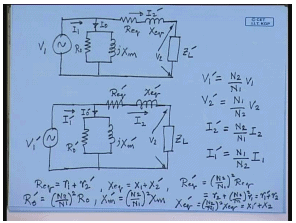For the primary we have already shown the approximate equivalent circuit to look like this; however, this can also be referred to the secondary side. They need to look like, where V 1 dash is equal to N 2 by N 1 into V 1. V 2 dash equal to N 1 by N 2 V 2. I 2 dash equal to N 2 by N 1 into I 2. I 1 dash equal to N 1 by N 2 into I 1. For the variables R e q dash R e q itself is R 1 plus R 2 dash. X e q equal to x 1 plus x 2 dash. R e q dash equal to N 2 by N 1 square R e q; hence, it is equal to R 2 plus N 2 by N 1 square R 1 equal to R 1 dash plus R 2.
Similarly, X e q dash equal to N 2 by N 1 square X e q equal to x 1 dash plus x 2. R 0 dash equal to N 2 by N 1 square R 0, and x m dash equal to N 2 by N 1 square x n. Therefore, in order to solve a given transformer problem the approximate equivalent circuit will normally be used. The approximate equivalent circuit can be either referred to the primary or referred to the secondary; the relationship between the variables and the equivalent circuit parameters are given by these equations.

Offer running on EduRev: Apply code STAYHOME200 to get INR 200 off on our premium plan EduRev Infinity!

66 docs|20 tests

,

,

,

,

,

,

,

,

,

,

,

,

,

,

,

,

,

,

,

,

,

;